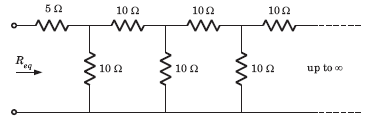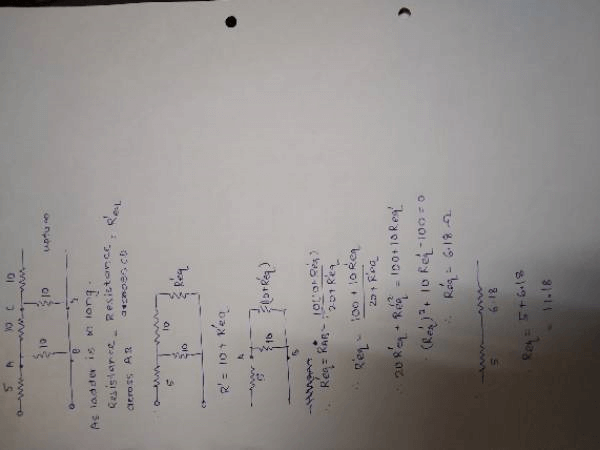Courses

# Infinite ladder GATE Notes | EduRev

## GATE : Infinite ladder GATE Notes | EduRev

The document Infinite ladder GATE Notes | EduRev is a part of GATE category.
All you need of GATE at this link: GATE

Req =  ?• a)
11.86 Ω
• b)
10 Ω
• c)
25 Ω
• d)
11.18 Ω

*As ladder is infinitely long. so choose repeating element and mark it as Req /Rnet.*
here choose 10 ohm- 10 ohm as repeating.
then connect that resistance in series with the element which chosen as Repeating ie across CD. Now solve first series combination
R1=(10 Req)
then connect that solved resistance in parallel across AB solve parallel combination
R2=(100 10Req)/(20 Req)
and equate with Req as (Resistance across AB=Resistance across CD).
Req=(100 10Req)/(20 Req)
solve this for Req you get quadratic equation
By solving we get Req=6.18ohm
Now solve the series combination to get final euivalant Resistance
final Req = 5 6.18=11.18 ohmsOffer running on EduRev: Apply code STAYHOME200 to get INR 200 off on our premium plan EduRev Infinity!

,

,

,

,

,

,

,

,

,

,

,

,

,

,

,

,

,

,

,

,

,

;